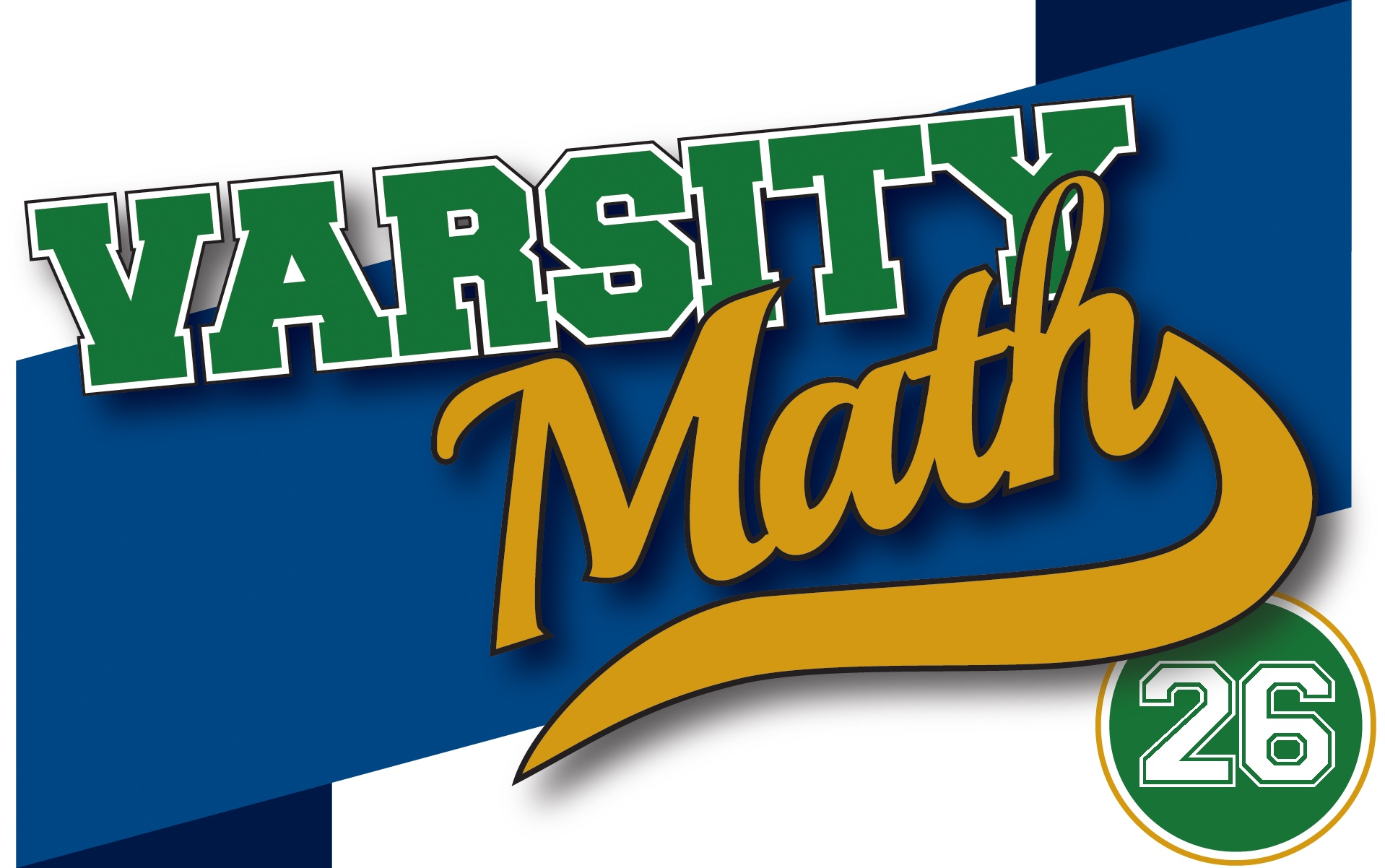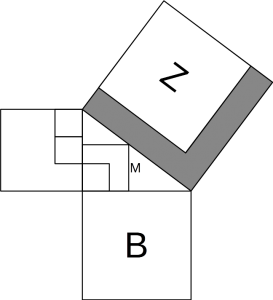The Varsity Math team is helping out at a local middle school math tournament, and the following two problems caught their eye. Both problems relate to the same diagram below, in which all of the quadrilaterals are squares and all of the smallest squares have side length equal to one unit.### HIJKMNOP

Assume squares B and Z are congruent.

What is the area of the shaded L-shaped region, in square units?

### Inscribed Square

Assume the area of square B is 16 square units.

What is the area of square M, in square units?

## Solutions to Week 25

Time to Burn. We can certainly time 60 minutes with one fuse, so a natural question is whether we can time 20 minutes with the other fuse. 20 is one third of 60, so is there a way to make the other fuse burn three times as fast? Intuitively, if it were always burning in three places, it would burn three times as fast, and so take only 20 minutes. So take the fuse, cut it into three pieces, and light each at one end. Since the fuse burns at a variable rate, it is unpredictable how long it will take each one to burn. But when one burns out, immediately cut the longer remaining fuse in half and light one end of the new piece. Continue in this fashion until the pieces are too small to deal with. You will have timed approximately 20 minutes.

To see this mathematically, suppose in the first step you have cut the fuse into pieces that take x < y < z minutes to burn, with x + y + z = 60. Then x < 20, and when that fuse has burnt out, there are y-x + z-x = 60 – 3x minutes of fuse left to burn. Those 60 – 3x minutes are again divided into three pieces x₁ < y₁ < z₁ with x₁ + y₁ + z₁ = 60 – 3x. So x₁ < 20 - x, and when that fuse has burnt out, there are (y₁ – x₁) + (z₁ – x₁) = 60 – 3(x + x₁) minutes of fuse remaining. Continuing in this way, those leftover pieces of fuse get shorter and shorter, and when they have burnt out completely, we have 0 minutes of fuse remaining with 0 = 60 – 3(x + x₁ + x₂ + …), so the total burn time of x + x₁ + x₂ + … is 20 minutes.

Therefore, you can time 60 minutes followed by 20 minutes for a total of 80 minutes.

Durable Digits. Suppose Quinn’s number is ABCD, all digits distinct. We must have A > D, since we know DCBA is smaller. So therefore when we subtract, we know we borrow from C, so that the last digit of the difference will be (10+D-A). Now there are two cases: (I) B ≤ C-1. In this case, we do not borrow in the tens digit subtraction, so the tens digit of the difference will be (C-1-B). And since B ≤ C-1, we also have B < C, so we do borrow in the hundreds digit, giving the answer (10+B-C) and finally (A-1-D) in the thousands place. When we reverse and add, we get (A-1-D)(10+B-C)(C-1-B)(10+D-A) + (10+D-A)(C-1-B)(10+B-C)(A-1-D) = 9999, which does not have four distinct digits. So we conclude that we are not in case (I), but rather case (II) B > C-1. Then we do borrow in the tens digit subtraction, yielding (9+C-B). Now we again split into two cases: (IIA) B-1 ≥ C. In this case we do not borrow in the hundreds place, yielding (B-1-C) and finally (A-D) in the thousands place. Reversing and adding gives (A-D)(B-1-C)(9+C-B)(10+D-A) + (10+D-A)(9+C-B)(B-1-C)(A-D) = 10890, which does have four different digits occurring in it, so we could be in case IIA. Finally, check case (IIB) B-1 > C. Then we borrow in the hundreds place, yielding (9+B-C) and (A-1-D) in the thousands place. Then reversing and adding would give (A-1-D)(9+B-C)(9+C-B)(10+D-A) + (10+D-A)(9+C-B)(9+B-C)(A-1-D) = 10989. So in either case, the digits A, B, C, and D, in no particular order, must be 0, 1, 8, and 9. Now it is not difficult to try various orders of these digits, noting that neither A nor D can be 0 and A must be bigger than D, to discover that 9108 – 8019 = 1089, which when reversed to 9801 and added gives 10890, is the only solution that satisfies all of the conditions. So Quinn’s number is 9108.

## Recent Weeks

Links to all of the puzzles and solutions are on the Complete Varsity Math page.

Come back next week for answers and more puzzles.